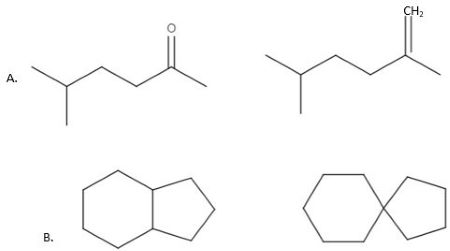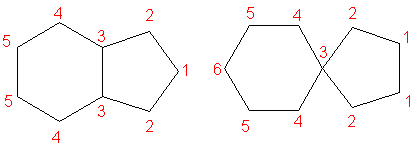# How would you use NMR (^1 H \;or\; ^{13}C) spectroscopy to distinguish between the following...

## Question:

How would you use {eq}NMR (^1 H \;or\; ^{13}C) {/eq} spectroscopy to distinguish between the following pairs of compounds?## NMR Spectroscopy

NMR spectroscopy is used when wanting to analyse organic molecules. Most commonly, we use {eq}^1H {/eq}-NMR and {eq}^{13}C {/eq}-NMR for organic molecules. The spectrum from each can give us an indication of what functional groups are present in the molecule and the number of electronically non-equivalent environments there are, that can help us distinguish between molecules.

A) the main different between the pairs in this part of the question is the first molecule has a carbonyl group (C=O) and the second has an alkene group (C=C). {eq}^1H {/eq}-NMR can be used to figure out the alkene group as we should see a signal around 4.5-6.5 ppm indicating a vinylic proton (C=C-H). {eq}^{13}C {/eq}-NMR can also be used but we would see a peak around 110-130 ppm for the C=C bond. For the ketone we would see a peak around 200-215 ppm indicating the presence of the C=O bond.

B) The two molecules in this part of the question do not differ in their functional groups, but differ in the number of environments, and therefore the number of signals on a spectrum, there are. Below we can see that the first molecule will give 5 environments on a {eq}^{13}C {/eq}-NMR spectrum, whereas the second will give 6. This number will be similar for the {eq}^1H {/eq}-NMR spectrum. We could consider the splitting of peaks for the {eq}^1H {/eq}-NMR spectrum, however by simply looking at the number of peaks we have already figured out which molecule is which.